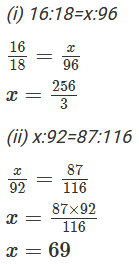×#### Thank you for registering.

One of our academic counsellors will contact you within 1 working day.

Click to Chat

1800-1023-196

+91-120-4616500

CART 0

• 0

MY CART (5)

Use Coupon: CART20 and get 20% off on all online Study Material

ITEM
DETAILS
MRP
DISCOUNT
FINAL PRICE
Total Price: Rs.

There are no items in this cart.
Continue Shopping• Complete JEE Main/Advanced Course and Test Series
• OFFERED PRICE: Rs. 15,900
• View Details

Chapter 9: Ratio and Proportion Exercise – 9.3

Question: 1

Which of the following are in proportion?

(i) 33, 44, 66, 88

(ii) 46, 69, 69, 46

(iii) 72, 84, 186, 217

Solution:Question: 2

Find x in the following proportions

(i) 16:18 = x: 96

(ii) x: 92 = 87: 116

Solution:Question: 3

The ratio of income to the expenditure of a family is 7: 6. Find the savings if the income is Rs.1400.

Solution:

The ratio of income and expenditure = 7: 6

7x = 1400

x = 200

Expenditure = 6x = 6 × 200 = Rs.1200

Savings = Income - Expenditure

= 1400 -1200

= Rs.200

Question: 4

The scale of a map is 1: 4000000. What is the actual distance between the two towns if they are 5cm apart on the map?

Solution:

The scale of map = 1: 4000000

Let us assume the actual distance between towns is x cm

1: 4000000 =5: x

x = 5 × 4000000 = 20000000 cm

1km = 1000 m

1m = 100 cm

Therefore

x = 200 km

Question: 5

The ratio of income of a person to his savings is 10: 1. If his savings for one year is Rs.6000, what is his income per month?

Solution:

The ratio of income of a person to his savings is 10: 1

Savings per month = 6000/12

= Rs.500

Then let income per month be x

x: 500 = 10:1

x = 500 × 10

x = 5000

Question: 6

An electric pole casts a shadow of length 20 metres at a time when a tree 6 metres high casts a shadow of length 8 metres. Find the height of the pole

Solution:

Height of the tree: Length of the shadow of tree

Height of the pole: Length of the shadow of pole

x: 20 = 6: 8

x = 120/8

x = 15### Course Features

• 728 Video Lectures
• Revision Notes
• Previous Year Papers
• Mind Map
• Study Planner
• NCERT Solutions
• Discussion Forum
• Test paper with Video Solution